# The Sharpe Ratio

## William F. Sharpe Stanford University

### Reprinted fromThe Journal of Portfolio Management, Fall 1994

This copyrighted material has been reprinted with permission from The Journal of Portfolio Management.
Copyright © Institutional Investor, Inc., 488 Madison Avenue, New York, N.Y. 10022,
a Capital Cities/ABC, Inc. Company. Phone (212) 224-3599.

. Over 25 years ago, in Sharpe , I introduced a measure for the performance of mutual funds and proposed the term reward-to-variability ratio to describe it (the measure is also described in Sharpe  ). While the measure has gained considerable popularity, the name has not. Other authors have termed the original version the Sharpe Index (Radcliff [1990, p. 286] and Haugen [1993, p. 315]), the Sharpe Measure (Bodie, Kane and Marcus [1993, p. 804], Elton and Gruber [1991, p. 652], and Reilly [1989, p.803]), or the Sharpe Ratio (Morningstar [1993, p. 24]). Generalized versions have also appeared under various names (see. for example, BARRA [1992, p. 21] and Capaul, Rowley and Sharpe [1993, p. 33]).

Bowing to increasingly common usage, this article refers to both the original measure and more generalized versions as the Sharpe Ratio. My goal here is to go well beyond the discussion of the original measure in Sharpe  and Sharpe , providing more generality and covering a broader range of applications.

### THE RATIO

Most performance measures are computed using historic data but justified on the basis of predicted relationships. Practical implementations use ex post results while theoretical discussions focus on ex ante values. Implicitly or explicitly, it is assumed that historic results have at least some predictive ability.

For some applications, it suffices for future values of a measure to be related monotonically to past values -- that is, if fund X had a higher historic measure than fund Y, it is assumed that it will have a higher future measure. For other applications the relationship must be proportional - - that is, it is assumed that the future measure will equal some constant (typically less than 1.0) times the historic measure.

To avoid ambiguity, we define here both ex ante and ex post versions of the Sharpe Ratio, beginning with the former. With the exception of this section, however, we focus on the use of the ratio for making decisions, and hence are concerned with the ex ante version. The important issues associated with the relationships (if any) between historic Sharpe Ratios and unbiased forecasts of the ratio are left for other expositions.

Throughout, we build on Markowitz' mean-variance paradigm, which assumes that the mean and standard deviation of the distribution of one-period return are sufficient statistics for evaluating the prospects of an investment portfolio. Clearly, comparisons based on the first two moments of a distribution do not take into account possible differences among portfolios in other moments or in distributions of outcomes across states of nature that may be associated with different levels of investor utility.

When such considerations are especially important, return mean and variance may not suffice, requiring the use of additional or substitute measures. Such situations are, however, beyond the scope of this article. Our goal is simply to examine the situations in which two measures (mean and variance) can usefully be summarized with one (the Sharpe Ratio).

#### The Ex Ante Sharpe Ratio

Let Rf represent the return on fund F in the forthcoming period and RB the return on a benchmark portfolio or security. In the equations, the tildes over the variables indicate that the exact values may not be known in advance. Define d, the differential return, as: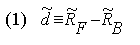Let d-bar be the expected value of d and sigmad be the predicted standard deviation of d. The ex ante Sharpe Ratio (S) is:In this version, the ratio indicates the expected differential return per unit of risk associated with the differential return.

#### The Ex Post Sharpe Ratio

Let RFt be the return on the fund in period t, RBt the return on the benchmark portfolio or security in period t, and Dt the differential return in period t: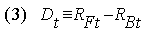Let D-bar be the average value of Dt over the historic period from t=1 through T: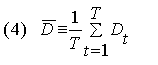and sigmaD be the standard deviation over the period 1: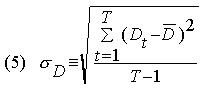The ex post, or historic Sharpe Ratio (Sh) is: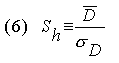In this version, the ratio indicates the historic average differential return per unit of historic variability of the differential return.

It is a simple matter to compute an ex post Sharpe Ratio using a spreadsheet program. The returns on a fund are listed in one column and those of the desired benchmark in the next column. The differences are computed in a third column. Standard functions are then utilized to compute the components of the ratio. For example, if the differential returns were in cells C1 through C60, a formula would provide the Sharpe Ratio using Microsoft's Excel spreadsheet program:

AVERAGE(C1:C60)/STDEV(C1:C60)

The historic Sharpe Ratio is closely related to the t-statistic for measuring the statistical significance of the mean differential return. The t-statistic will equal the Sharpe Ratio times the square root of T (the number of returns used for the calculation). If historic Sharpe Ratios for a set of funds are computed using the same number of observations, the Sharpe Ratios will thus be proportional to the t-statistics of the means.

#### Time Dependence

The Sharpe Ratio is not independent of the time period over which it is measured. This is true for both ex ante and ex post measures.

Consider the simplest possible case. The one-period mean and standard deviation of the differential return are, respectively, d-bar1 and sigmad1. Assume that the differential return over T periods is measured by simply summing the one-period differential returns and that the latter have zero serial correlation. Denote the mean and standard deviation of the resulting T-period return, respectively, d-barT and sigmadT. Under the assumed conditions: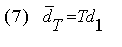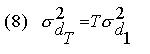and:Letting S1 and ST denote the Sharpe Ratios for 1 and T periods, respectively, it follows that: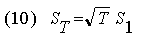In practice, the situation is likely to be more complex. Multiperiod returns are usually computed taking compounding into account, which makes the relationship more complicated. Moreover, underlying differential returns may be serially correlated. Even if the underlying process does not involve serial correlation, a specific ex post sample may.

It is common practice to "annualize" data that apply to periods other than one year, using equations (7) and (8). Doing so before computing a Sharpe Ratio can provide at least reasonably meaningful comparisons among strategies, even if predictions are initially stated in terms of different measurement periods.

To maximize information content, it is usually desirable to measure risks and returns using fairly short (e.g. monthly) periods. For purposes of standardization it is then desirable to annualize the results.

To provide perspective, consider investment in a broad stock market index, financed by borrowing. Typical estimates of the annual excess return on the stock market in a developed country might include a mean of 6% per year and a standard deviation of 15%. The resulting excess return Sharpe Ratio of "the stock market", stated in annual terms would then be 0.40.

#### Correlations

The ex ante Sharpe Ratio takes into account both the expected differential return and the associated risk, while the ex post version takes into account both the average differential return and the associated variability. Neither incorporates information about the correlation of a fund or strategy with other assets, liabilities, or previous realizations of its own return. For this reason, the ratio may need to be supplemented in certain applications. Such considerations are discussed in later sections.

#### Related Measures

The literature surrounding the Sharpe Ratio has, unfortunately, led to a certain amount of confusion. To provide clarification, two related measures are described here. The first uses a different term to cover cases that include the construct that we call the Sharpe Ratio. The second uses the same term to describe a different but related construct.

Whether measured ex ante or ex post, it is essential that the Sharpe Ratio be computed using the mean and standard deviation of a differential return (or, more broadly, the return on what will be termed a zero investment strategy). Otherwise it loses its raison d'etre. Clearly, the Sharpe Ratio can be considered a special case of the more general construct of the ratio of the mean of any distribution to its standard deviation.

In the investment arena, a number of authors associated with BARRA (a major supplier of analytic tools and databases) have used the term information ratio to describe such a general measure. In some publications , the ratio is defined to apply only to differential returns and is thus equivalent to the measure that we call the Sharpe Ratio (see, for example, Rudd and Clasing [1982, p. 513] and Grinold [1989, p. 31]). In others, it is also encompasses the ratio of the mean to the standard deviation of the distribution of the return on a single investment, such as a fund or a benchmark (see, for example, BARRA [1993, p. 22]). While such a "return information ratio" may be useful as a descriptive statistic, it lacks a number of the key properties of what might be termed a "differential return information ratio" and may in some instances lead to wrong decisions.

For example, consider the choice of a strategy involving cash and one of two funds, X and Y. X has an expected return of 5% and a standard deviation of 10%. Y has an expected return of 8% and a standard deviation of 20%. The riskless rate of interest is 3%. According to the ratio of expected return to standard deviation, X (5/10, or 0.50) is superior to Y (8/20, or 0.40). According to the Sharpe Ratios using excess return, X (2/10, or 0.20) is inferior to Y (5/20, or 0.25).

Now, consider an investor who wishes to attain a standard deviation of 10%. This can be achieved with fund X, which will provide an expected return of 5.0%. It can also be achieved with an investment of 50% of the investor's funds in Y and 50% in the riskless asset. The latter will provide an expected return of 5.5% -- clearly the superior alternative.

Thus the Sharpe Ratio provides the correct answer (a strategy using Y is preferred to one using X), while the "return information ratio" provides the wrong one.

In their seminal work, Treynor and Black , defined the term "Sharpe Ratio" as the square of the measure that we describe. Others, such as Rudd and Clasing [1982, p. 518] and Grinold [1989, p. 31], also use such a definition.

While interesting in certain contexts, this construct has the curious property that all values are positive -- even those for which the mean differential return is negative. It thus obscures important information concerning performance. We prefer to follow more common practice and thus refer to the Treynor-Black measure as the Sharpe Ratio squared (SR2). 2:

We focus here on the Sharpe Ratio, which takes into account both risk and return without reference to a market index. [Sharpe 1966, 1975] discusses both the Sharpe Ratio and measures based on market indices, such as Jensen's alpha and Treynor's average excess return to beta ratio.

### Scale Independence

Originally, the benchmark for the Sharpe Ratio was taken to be a riskless security. In such a case the differential return is equal to the excess return of the fund over a one-period riskless rate of interest. Many of the descriptions of the ratio in Sharpe [1966, 1975] focus on this case .

More recent applications have utilized benchmark portfolios designed to have a set of "factor loadings" or an "investment style" similar to that of the fund being evaluated. In such cases the differential return represents the difference between the return on the fund and the return that would have been obtained from a "similar" passive alternative. The difference between the two returns may be termed an "active return" or "selection return", depending on the underlying procedure utilized to select the benchmark.

Treynor and Black  cover the case in which the benchmark portfolio is, in effect, a combination of riskless securities and the "market portfolio". Rudd and Clasing  describe the use of benchmarks based on factor loadings from a multifactor model. Sharpe  uses a procedure termed style analysis to select a mix of asset class index funds that have a "style" similar to that of the fund. When such a mix is used as a benchmark, the differential return is termed the fund's selection return. The Sharpe Ratio of the selection return can then serve as a measure of the fund's performance over and above that due to its investment style. 3:

Central to the usefulness of the Sharpe Ratio is the fact that a differential return represents the result of a zero-investment strategy. This can be defined as any strategy that involves a zero outlay of money in the present and returns either a positive, negative or zero amount in the future, depending on circumstances. A differential return clearly falls in this class, since it can be obtained by taking a long position in one asset (the fund) and a short position in another (the benchmark), with the funds from the latter used to finance the purchase of the former.

In the original applications of the ratio, where the benchmark is taken to be a one- period riskless asset, the differential return represents the payoff from a unit investment in the fund, financed by borrowing. 4: More generally, the differential return corresponds to the payoff obtained from a unit investment in the fund, financed by a short position in the benchmark. For example, a fund's selection return can be considered to be the payoff from a unit investment in the fund, financed by short positions in a mix of asset class index funds with the same style.

A differential return can be obtained explicitly by entering into an agreement in which a party and a counterparty agree to swap the return on the benchmark for the return on the fund and vice-versa. A forward contract provides a similar result. Arbitrage will insure that the return on such a contract will be very close to the excess return on the underlying asset for the period ending on the delivery date. 5: A similar relationship holds approximately for traded contracts such as stock index futures , which clearly represent zero-investment strategies. 6:

To compute the return for a zero-investment strategy the payoff is divided by a notional value. For example, the dollar payoff for a swap is often set to equal the difference between the dollar return on an investment of \$X in one asset and that on an investment of \$X in another. The net difference can then be expressed as a proportion of \$X, which serves as the notional value. Returns on futures positions are often computed in a similar manner, using the initial value of the underlying asset as a base. In effect, the same approach is utilized when the difference between two returns is computed.

Since there is zero net investment in any such strategy, the percent return can be made as large or small as desired by simply changing the notional value used in such a computation. The scale of the return thus depends on the more- or-less arbitrary choice of the notional value utilized for its computation. 7:

Changes in the notional value clearly affect the mean and the standard deviation of the distribution of return, but the changes are of the same magnitude, leaving the Sharpe Ratio unaffected. The ratio is thus scale independent. 8:

### The Influence of a Zero Investment Strategy on Asset Risk and Return

Scale independence is more than a mathematical artifact. It is key to understanding why the Sharpe Ratio can provide an efficient summary statistic for a zero- investment strategy. To show this, we consider the case of an investor with a pre-existing portfolio who is considering the choice of a zero investment strategy to augment current investments.

#### The Relative Position in a Zero Investment Strategy

Assume that the investor has \$A in assets and has placed this money in an investment portfolio with a return of RI. She is considering investment in a zero-investment strategy that will provide a return of d per unit of notional value. Denote the notional value chosen as V (e.g. investment of V in a fund financed by a short position of V in a benchmark). Define the relative position, p, as the ratio of the notional value to the investor's assets: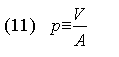The end-of-period payoff will be: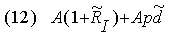Let RA denote the total return on the investor's initial assets. Then: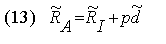If R-barA denotes the expected return on assets and R- barI the expected return on the investment:Now, let sigmaA, sigmaI and sigmad denote the standard deviations of the returns on assets, the investment and the zero-investment strategy, respectively, and rhoId the correlation between the return on the investment and the return on the zero-investment strategy. Then: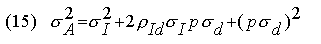or, rewriting slightly: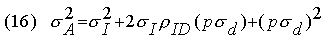#### The Risk Position in a Zero Investment Strategy

The parenthesized expression (p sigmad) is of particular interest. It indicates the risk of the position in the zero-investment strategy relative to the investor's overall assets. Let k denote this risk positionFor many purposes it is desirable to consider k as the relevant decision variable. Doing so states the magnitude of a zero-investment strategy in terms of its risk relative to the investor's overall assets. In effect, one first determines k, the level of risk of the zero- investment strategy. Having answered this fundamental question, the relative (p) and absolute (V) amounts of notional value for the strategy can readily be determined, using equations (17) and (11). 9:

#### Asset Risk and Expected Return

It is straightforward to determine the manner in which asset risk and expected return are related to the risk position of the zero investment strategy, its correlation with the investment, and its Sharpe Ratio.

Substituting k in equation (16) gives the relationship between 1) asset risk and 2) the risk position and the correlation of the strategy with the investment: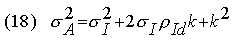To see the relationship between asset expected return and the characteristics of the zero investment strategy, note that the Sharpe Ratio is the ratio of d-bar to sigmad. It follows thatSubstituting equation (19) in equation (14) gives: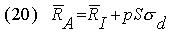or: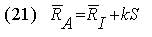which shows that the expected return on assets is related directly to the product of the risk position times the Sharpe Ratio of the strategy.

By selecting an appropriate scale, any zero investment strategy can be used to achieve a desired level (k) of relative risk. This level, plus the strategy's Sharpe Ratio, will determine asset expected return, as shown by equation (21). Asset risk, however, will depend on both the relative risk (k) and the correlation of the strategy with the other investment (rhoId ). In general, the Sharpe Ratio, which does not take that correlation into account, will not by itself provide sufficient information to determine a set of decisions that will produce an optimal combination of asset risk and return, given an investor's tolerance of risk.

### Adding a Zero-Investment Strategy to an Existing Portfolio

Fortunately, there are important special cases in which the Sharpe Ratio will provide sufficient information for decisions on the optimal risk/return combination: one in which the pre-existing portfolio is riskless, the other in which it is risky.

#### Adding a Strategy to a Riskless Portfolio

Suppose first that an investor plans to allocate money between a riskless asset and a single risky fund (e.g. a "balanced" fund). This is, in effect, the case analyzed in Sharpe [1966,1975].

We assume that there is a pre-existing portfolio invested solely in a riskless security, to which is to be added a zero investment strategy involving a long position in a fund, financed by a short position in a riskless asset (i.e., borrowing). Letting Rc denote the return on such a "cash equivalent", equations (1) and (13) can be written as:andSince the investment is riskless, its standard deviation of return is zero, so both the first and second terms on the right-hand side of equation (18) become zero, giving: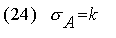The investor's total risk will thus be equal to that of the position taken in the zero investment strategy, which will in turn equal the risk of the position in the fund.

Letting SF represent the Sharpe Ratio of fund F, equation (21) can be written: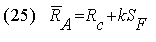It is clear from equations (24) and (25) that the investor should choose the desired level of risk (k), then obtain that level of risk by using the fund (F) with the greatest excess return Sharpe Ratio. Correlation does not play a role since the remaining holdings are riskless.

This is illustrated in the Exhibit. Points X and Y represent two (mutually exclusive) strategies. The desired level of risk is given by k. It can be obtained with strategy X using a relative position of px (shown in the figure at point PxX) or with strategy Y using a relative position of pY (shown in the figure at point PyY). An appropriately-scaled version of strategy X clearly provides a higher mean return (shown at point MRx) than an appropriately-scaled version of strategy Y (shown at point MRy). Strategy X is hence to be preferred.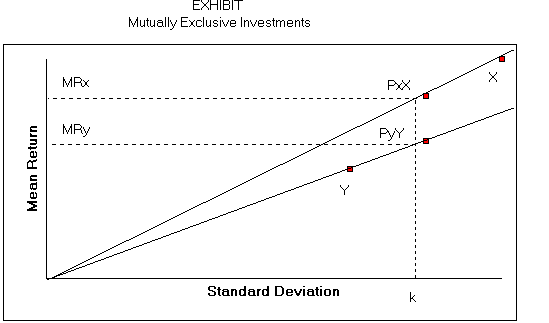The Exhibit shows that the mean return associated with any desired risk position will be greater if strategy X is adopted instead of strategy Y. But the slope of such a line is the Sharpe Ratio. Hence, as long as only the mean return and the risk position of the zero-investment strategy are relevant, the optimal solution involves maximization of the Sharpe Ratio of the zero-investment strategy.

Consider, for example, a choice between fund XX, with a risk of 10% and an excess return Sharpe Ratio of 0.20 and fund YY with a risk of 20% and an excess return Sharpe Ratio of 0.25. Assume the investor has \$100 to invest and desires a level of risk (here, k) equal to 15%.

The optimal strategy involves investment of \$100 in the riskless asset plus a zero-investment strategy based on fund YY. To make the risk of the latter equal to 15%, a relative position (p) of 0.75 should be taken. This, in turn, requires an investment of \$75 in the fund, financed by \$75 of borrowing (i.e. a short position in the riskless asset). The net position in the riskless asset will thus be \$25 (\$100 - \$75), with \$75 invested in Fund YY.

In this case the investor's tasks include the selection of the fund with the greatest Sharpe Ratio and the allocation of wealth between this fund and borrowing or lending, as required to obtain the desired level of asset risk.

#### Adding a Strategy to a Risky Portfolio

Consider now the case in which a single fund is to be selected to complement a pre-existing group of risky investments. For example, an investor might have \$100, with \$80 already committed (e.g. to a group of bond and stock funds). The goal is to allocate the remaining \$20 between a riskless asset ("cash") and a single risky fund (e.g. a "growth stock fund"), accepting the possibility that the amount allocated to cash might be positive, zero or negative, depending on the desired risk and the risk of the chosen fund.

In this case the investment should be taken as the pre-existing investment plus a riskless asset (in the example, \$80 in the initial investments plus \$20 in cash equivalents). The return on this total portfolio will be RI. The zero- investment strategy will again involve a long position in a risky fund and a short position in the riskless asset.

As stated earlier, in such a case it will not necessarily be optimal to select the fund with the largest possible Sharpe Ratio. While the ratio takes into account two key attributes of the predicted performance of a zero-investment strategy (its expected return and its risk), it does not include information about the correlation of its return with that of the investor's other holdings (rhoId). It is entirely possible that a fund with a smaller Sharpe Ratio could have a sufficiently smaller correlation with the investor's other assets that it would provide a higher expected return on assets for any given level of overall asset risk.

However, if the alternative funds being analyzed have similar correlations with the investor's other assets, it will still be optimal to select the fund with the greatest Sharpe Ratio. To see this, note that with rhoId taken as given, equation (18) shows that there is a one-to-one correspondence between sigmaA and k. Thus, for any desired level of asset risk, the investor chooses the corresponding risk position k given by equation (18), regardless of the fund to be employed.

But, as before, the expected return on assets will be:which can be maximized by selecting the fund with the largest Sharpe Ratio.

The practical implication is clear. When choosing one from among a group of funds of a particular type for inclusion in a larger set of holdings, the one with the largest predicted excess return Sharpe Ratio may reasonably be chosen, if it can be assumed that all the funds in the set have similar correlations with the other holdings. If this condition is not met, some account should be taken of the differential levels of such correlations.

#### The Choice of a Set of Uncorrelated Strategies

Suppose finally that an investor has a pre-existing set of investments and is considering taking positions in one or more zero-investment strategies, each of which is uncorrelated both with the existing investments and with each of the other such strategies. Such lack of correlation is generally assumed for residual returns from an assumed factor model and hence applies to strategies in which long and short positions are combined to obtain zero exposures to all underlying factors in such a model.

In particular, this is assumed to hold for the "non-market returns" which are the residual returns in one-factor "market models" of the type employed in Treynor-Black . It is also assumed to hold for the "active returns" that constitute the residual returns in a model of the type used by BARRA (described, for example, in Grinold ).

Most germane, perhaps, for selecting funds, this is assumed to hold for the "selection returns" that constitute the residuals from the asset class factor model used in the style analysis procedure described in 10:

Under the assumed conditions, the counterpart to equation (13) is: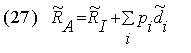where pi represents the relative position taken in strategy i and di represents its return.

Letting sigmadi represent the risk of position i, asset risk is given by: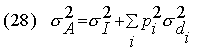and expected asset return by:Adding subscriptions to equations (21) and (18), and substituting the results gives: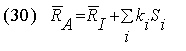and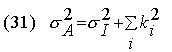Now, assume that the investor's goal is to maximize a standard risk- adjusted expected return of the form: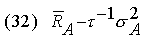where tau represents risk tolerance (the marginal rate of substitution of variance for expected return). Substituting equations (30) and (31) in (32) gives:Since the terms involving the initial investment will be unaffected by the decisions (ki's) concerning the zero investment strategies, it suffices to maximize: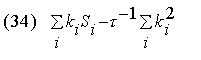To do so, the partial derivative with respect to each decision variable (ki) should be set to zero: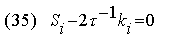The optimal risk position in strategy i is thus:Hence the risk levels of the strategies should be proportional to their Sharpe Ratios. Strategies with zero predicted Sharpe Ratios should be ignored. Those with positive ratios should be "held long", and those with negative ratios "held short". If strategy X has a positive Sharpe Ratio that is twice as large as that of strategy Y, twice as much risk should be taken with X as with Y. The overall scale of all the positions should, in turn, be proportional to the investor's risk tolerance.

An interesting application occurs when long and short positions can be taken (e.g. via financial futures) in the asset classes that underlie a style analysis model of the type described in Sharpe . In principle, funds should be selected based only on their selection returns, with the respective amounts of selection risk set in proportion to the funds' selection return Sharpe Ratios. The net exposures to asset classes required to implement this mixture of zero investment strategies can then be compared with the investor's desired passive asset mix to determine needed net positions.

### Summary

The Sharpe Ratio is designed to measure the expected return per unit of risk for a zero investment strategy. The difference between the returns on two investment assets represents the results of such a strategy. The Sharpe Ratio does not cover cases in which only one investment return is involved.

Clearly, any measure that attempts to summarize even an unbiased prediction of performance with a single number requires a substantial set of assumptions for justification. In practice, such assumptions are, at best, likely to hold only approximately. Certainly, the use of unadjusted historic (ex post) Sharpe Ratios as surrogates for unbiased predictions of ex ante ratios is subject to serious question. Despite such caveats, there is much to recommend a measure that at least takes into account both risk and expected return over any alternative that focuses only on the latter.

For a number of investment decisions, ex ante Sharpe Ratios can provide important inputs. When choosing one from among a set of funds to provide representation in a particular market sector, it makes sense to favor the one with the greatest predicted Sharpe Ratio, as long as the correlations of the funds with other relevant asset classes are reasonably similar. When allocating funds among several such funds, it makes sense to allocate funds such that the selection (residual) risk levels are proportional to the predicted Sharpe Ratios for the selection (residual) returns. If some of the implied net positions are infeasible or involve excessive transactions costs, of course, the decision rules must be modified. Nonetheless, Sharpe Ratios may still provide useful guidance.

Whatever the application, it is essential to remember that the Sharpe Ratio does not take correlations into account. When a choice may affect important correlations with other assets in an investor's portfolio, such information should be used to supplement comparisons based on Sharpe Ratios.

All the same, the ratio of expected added return per unit of added risk provides a convenient summary of two important aspects of any strategy involving the difference between the return of a fund and that of a relevant benchmark. The Sharpe Ratio is designed to provide such a measure. Properly used, it can improve the process of managing investments.

### Endnotes

1. We use the formula for the standard deviation of a population, taking the observations as a sample. For applications in which the value of T is the same for all the funds being measured, the standard deviation of the historic data (in which the denominator is T rather than T-1) can generally be used instead, since the relative magnitudes of the resulting measures would be the same.

2. Treynor and Black showed that if resources are allocated optimally, the SR2 of a portfolio will equal the sum of the SR2 values for its components. This follows from the fact that the optimal holding of a component will be proportional to the ratio of its mean differential return to the square of the standard deviation of its differential return. Thus, for example, components with negative means should be held in negative amounts. In this context, the product of the mean return and the optimal holding will always be positive. For completeness, it should be noted that Treynor and Black used the term appraisal ratio to refer to what we term here the SR2 of a component and the term Sharpe Ratio to refer to the SR2 of the portfolio, although other authors have used the latter term for both the portfolio and its components.

3. This type of application is described in BARRA [1992, p. 21].

4. In this context, maximization of the Sharpe Ratio is the normative equivalent to the separation theorem first put forth in Tobin  in a positive context.

5. To see this, note that by borrowing money to purchase the underlying asset, one can obtain precisely the same asset at the delivery date. The ending value of such a strategy will be perfectly correlated with the value of the forward contract and neither will require any outlay. If the payoffs at the end of the period differ, one could take a long position in one combination (e.g. the forward contract or the asset/borrowing combination) and a short position in the other and obtain a guaranteed payment at the end of the period with no outlay at any other time. This is unlikely to be the case in a market populated by astute investors. In practice, transactions costs will limit the precision of the relationship.

6. Futures contracts are often not protected against changes in value due to (for example) dividend payments. They also generally require daily marking to market. For these reasons they differ from forward contracts with dividend protection, for which the arbitrage relationship will hold within the bounds of transactions costs. Futures contracts generally require that margin be posted. However, this is not an investment in the underlying asset.

7. Despite this drawback, once a notional value has been selected, the actual rate of return can be used for comparison purposes.

8. Indeed, a Sharpe Ratio can be computed without regard to notional value by simply using the mean and standard deviation of the distribution of the final payoff.

9. To see the advantages of concentration on the risk position of a strategy, consider two funds. One (X) invests directly, the other (Y) borrows money at the riskless rate and invests in X, with a leverage ratio of 2 to 1. Let kx be the optimal position in fund X. Clearly the optimal position in fund Y will be half as large. However, the standard deviation of return on fund Y will be twice that of fund X. Thus the optimal risk position in Y will be the same as that in X.

10. In fact, the basic relationship on which this section builds was first obtained by Treynor and Black .

### References

BARRA Newsletter, September/October 1992, May/June 1993, BARRA, Berkeley, Ca.

Bodie, Zvi, Alex Kane and Alan J. Marcus. Investments, 2d edition. Homewood, IL: Richard D. Irwin, 1993.

Capaul, Carlo, Ian Rowley, and William F. Sharpe. "International Value and Growth Stock Returns," Financial Analysts Journal, January/February 1993, pp. 27-36.

Elton, Edwin J., and Martin J. Gruber. Modern Portfolio Theory and Investment Analysis, 4th edition. New York: John Wiley & Sons, 1991.

Grinold, Richard C. "The Fundamental Law of Active Management," Journal of Portfolio Management, Spring 1989, pp. 30-37.

Haugen, Robert A. Modern Investment Theory, 3d edition. Englewood Cliffs, NJ: Prentice-Hall, 1993.

"Morningstar Mutual Funds User's Guide." Chicago: Morningstar Inc., 1993.

Radcliff, Robert C. Investment Concepts, Analysis, Strategy, 3d edition. New York: HarperCollins, 1990.

Reilly, Frank K. Investment Analysis and Portfolio Management, 3d edition. Chicago: The Dryden Press, 1989.

Rudd, Andrew, and Henry K. Clasing. Modern Portfolio Theory, The Principles of Investment Management. Homewood, IL: Dow-Jones Irwin, 1982.

Sharpe, William F. "Mutual Fund Performance." Journal of Business, January 1966, pp. 119-138.

-----. "Adjusting for Risk in Portfolio Performance Measurement." Journal of Portfolio Management, Winter 1975, pp. 29-34.

-----. "Asset allocation: Management Style and Performance Measurement," Journal of Portfolio Management, Winter 1992, pp. 7-19.

Tobin, James. "Liquidity Preference as Behavior Toward Risk." Review of Economic Studies, February 1958, pp. 65-86.

Treynor, Jack L., and Fischer Black. "How to Use Security Analysis to Improve Portfolio Selection." Journal of Business, January 1973, pp. 66-85.